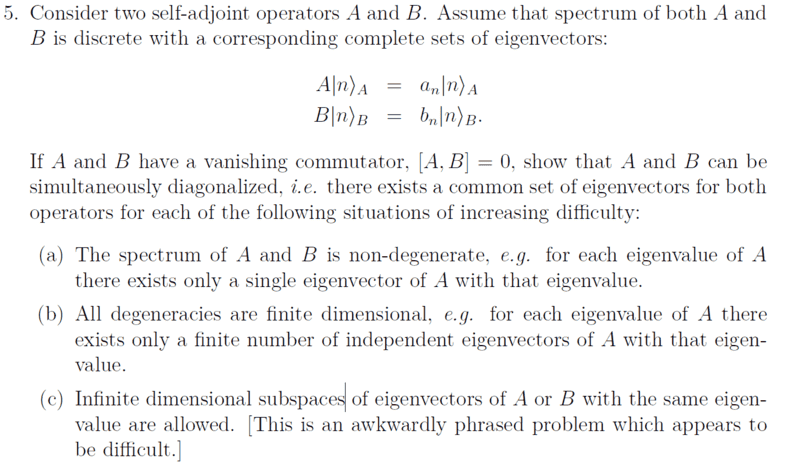# Simultaneous Diagonalization for Two Self-Adjoint Operators

• LarryC
In summary, the author is questioning the validity of C, which is a more traditional way of thinking about infinite dimensionality. C is based on the idea that all vectors in an infinite dimension space have the same eigenvalue, which is different from A and B which are based on the idea that there can be different vectors with the same eigenvalue.

#### LarryC(a) and (b) are fairly traditional, but I have trouble understanding the phrasing of (c). What makes the infinite dimensionality in (c) different from (a) and (b)?

#### Attachments

B and C appear contradictory at least the way I read it.

An example of C is take a Hilbert Space and the identity operator. All vectors have the same eigenvalue ie 1 and any orthogonal infinite basis generates a subspace with the same eigenvalue. If this is beginning level linear algebra I think the author of the problem needs to think a bit harder about appropriate questions to ask and its wording. I know finite dimensional linear algebra well, Hilbert Spaces, and even Rigged Hilbert Spaces and would never ask a question like that.

My suggestion is take it along to a tutorial or see your lecturer about it.

Thanks
Bill

LarryC said:
(a) and (b) are fairly traditional, but I have trouble understanding the phrasing of (c). What makes the infinite dimensionality in (c) different from (a) and (b)?
Show us your proof for part (b). There's probably a step in there that doesn't necessarily hold for infinite dimension subspaces.

•bhobba
vela said:
Show us your proof for part (b). There's probably a step in there that doesn't necessarily hold for infinite dimension subspaces.

Yes B is not that hard for finite dimensional spaces - as a hint just look into the spectral theorem. I can write the proof in a few lines using bra-ket notation. Extending that to infinite dimensional spaces is much more difficult (it requires the so called Nuclear Spectral theorem - also called the Gelfand-Maurin theorem) which may be the purpose of the question - infinite dimensional spaces are problematical.

Added later - if you are still stuck then under OCW MIT has a full analysis of this problem. It must be from a standard textbook - maybe Griffiths or something like that otherwise they probably would not have posted a write up on it. So Google is your friend here - and the answer is not 100^% what the question would imply - but its in your court to now. If gets around the infinite dimensional case by using infinite matrices - sneaky - but maybe not quite rigorous.

Thanks
Bill

Last edited:
The OP seems to have left the thread. How to locate the full answer has been posted, as well some advanced issues with infinite spaces. It looks like it has run its course so will be closed. As always if someone wants to continue drop a line to a mentor, but for now it will remain closed.

Thanks
Bill